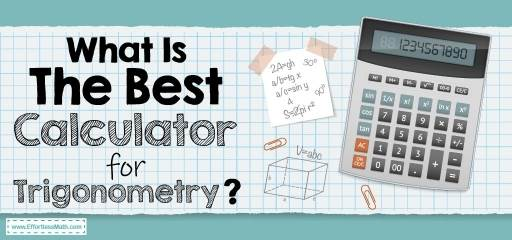# What Is The Best Calculator For Trigonometry?Trigonometry can be a challenging subject, and you need specific functions in a calculator to solve such problems. That is why you need the best calculator that will make solving trigonometric expressions easier.

While there are limited options, here are the top ones you can choose from for trigonometry.

• 1- TI-84 Plus CE
• 2- fx-115ESPLUS2
• 3- PRIZM FX-CG50
• 4- TI-36X Pro

The Absolute Best Book to Ace Trigonometry

## Texas Instruments TI-84 Plus CE

Texas Instruments is one of the best brands known for its excellent scientific calculators.

The TI-84 Plus is the ideal choice for trigonometry as it is a graphing calculator and has all the necessary features to solve complex trigonometry questions.

The calculator is also approved to use in IB exams.

Here are the top features of this calculator:

• Ideal to use for AP, SAT, and PSAT tests
• High-resolution readability for ease of use
• Perfect to use for Trigonometry, Calculus, and much more
• Ideal trigonometry calculator for beginners

## Casio fx-115ESPLUS2 2nd Edition

The Casio fx-115ESPLUS2 2nd Edition is allowed for use in the SAT and PSAT, and it has all the features you need to solve linear regression, complex calculations, basic arithmetic, and much more.

The calculator is easy to use, and you can also use it for fort metric conversions. It has all the features you need to solve trigonometric expressions in no time.

Here are the top features of this calculator:

• Over 280 features
• Multi-relay features that allow you to check all the steps, edit, and recalculate a problem
• It can be used for Calculus, Trigonometry, Algebra, Statistics, and much more
• Multi-line display

## CASIO PRIZM FX-CG50 Color Graphing Calculator

Another Casio calculator that is best to use for Trigonometry, Calculus, Physics, and much more.

The Prizm technology will allow you to plot graphs in colors, and the calculator offers real images for ease of learning.

Here are the top features of this calculator:

• PC link port to transfer data from the computer
• Memory storage of 16MB
• Natural textbook display

## Texas Instruments TI-36X Pro

The TI-36X is ideal for all students, be it, students in junior high or college students.

You can use this calculator for various subjects such as trigonometry, calculus, statistics, algebra, and much more.

Remember that this is not a graphing calculator, which is why if you need a graphing calculator, you can choose from others on our list.

Here are the top features of this calculator:

• Vector and matrix entry window
• Four-line display for comprehensive calculations
• Display defined functions in tabular forms
• MultiView display for showing multiple calculations

## Final Words

The trigonometry calculator is perfect for you depending on your preferences and needs. These four calculators are the best trigonometry calculators you can choose from and solve calculations in no time. There are graphing and non-graphing variants to help you make the right choice.

So, what are you waiting for then? Please choose the best trigonometry calculator from our list and see how easy it becomes for you to solve complex equations in no time.

The Best Books to Ace the Trigonometry Test

### What people say about "What Is The Best Calculator For Trigonometry? - Effortless Math: We Help Students Learn to LOVE Mathematics"?

No one replied yet.

X
51% OFF

Limited time only!

Save Over 51%

SAVE \$15

It was \$29.99 now it is \$14.99